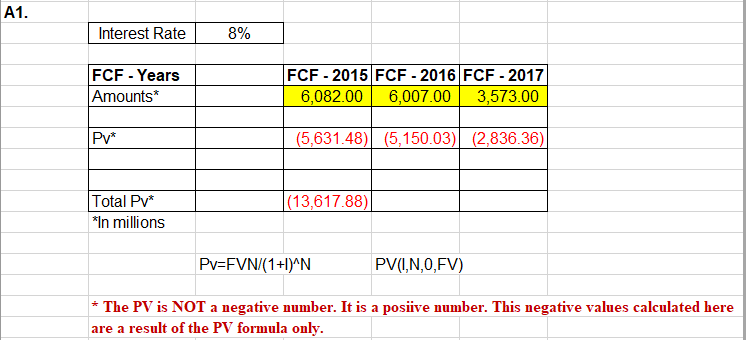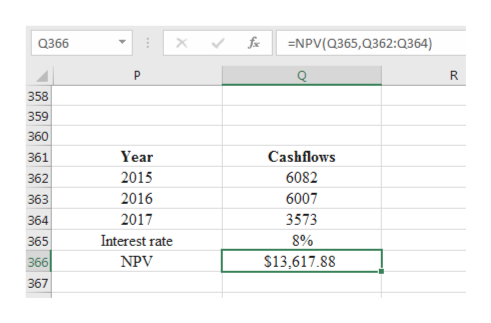# A1.Interest Rate8%FCF - 2015 FCF -2016 FCF -20176,082.00FCF - YearsAmounts*6,007.003,573.00Pv*(5,631.48)(5,150.03) (2,836.36)Total Pv*|(13,617.88)*In millionsPv=FVN/(1+I)^NPV(,N,0,FV)The PV is NOT a negative number. It is a posiive number. This negative values calculated hereare a result of the PV formula only

Question
9 views

Which figure in this worksheet is the Present Value of the company in 2014?

* *****I think it is

 (13,617.88)help_outlineImage TranscriptioncloseA1. Interest Rate 8% FCF - 2015 FCF -2016 FCF -2017 6,082.00 FCF - Years Amounts* 6,007.00 3,573.00 Pv* (5,631.48)(5,150.03) (2,836.36) Total Pv* |(13,617.88) *In millions Pv=FVN/(1+I)^N PV(,N,0,FV) The PV is NOT a negative number. It is a posiive number. This negative values calculated here are a result of the PV formula only fullscreen
check_circle

star
star
star
star
star
1 Rating
Step 1

The present value is calculated today to determine the present value of future cashflows at the given discount rate.

Step 2

Given:

Interest rate is 8%

Future cash flows for three years are as follows:

Step 3

Explanation:

By using the excel sheet, the calculated NPV for the year 2014...help_outlineImage Transcriptionclosefoix NPV(Q365,Q362:Q364) Q366 P R. 358 359 360 Year Cashflows 361 2015 6082 362 6007 2016 363 2017 3573 364 Interest rate 8% 365 366 \$13,617.88 NPV 367 fullscreen

### Want to see the full answer?

See Solution

#### Want to see this answer and more?

Solutions are written by subject experts who are available 24/7. Questions are typically answered within 1 hour.*

See Solution
*Response times may vary by subject and question.
Tagged in